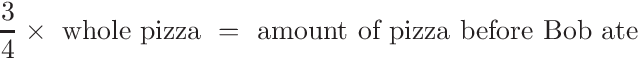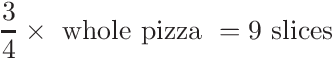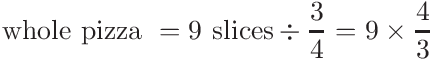# Why does the number of slices present before bob came divided by 3/4 equal the total number of slices in the pizza?

• This post is deleted!

• [Originally posted in Discussions]

Why does the number of slices present before bob came divided by 3/4 (how much of the pizza it was) the total number of slices in the pizza? How does that work? Shouldn't you do some more? I don't get it.

• This is becauseand we found that there were 9 slices before Bob ate (based on working backwards from the 3 slices at the end).To find the number of slices in the original pizza, divide both sides by 3/4:Let me know if you have any more questions!

Happy Learning!

The Daily Challenge Team

• [Originally posted in Discussions]

But why do you divide?

• You divide because in the original equation in my prior post, the "number of slices in the whole pizza" is multiplied by 3/4:We want to solve for the number of slices in the whole pizza, so we want to get the "whole pizza" by itself. That is why we divide by 3/4.

I hope this helps!

Happy Learning,

The Daily Challenge Team